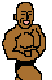## Mira-type stars: Multiharmonic analysis

(posted by I. Andronov, vsnet 860)

\documentstyle[11pt]{article}
\textwidth 170mm
\textheight 230mm
\oddsidemargin -3mm
\topmargin -16mm
\begin{document}
\begin{center}


{\Large\bf MULTIHARMONIC ANALYSIS OF MIRA--TYPE STARS} \footnote{IAU Symposium 180 "Planetary Nebulae" Poster I-8}\\[1mm] L.S. Kudashkina, I.L. Andronov\\[1mm] Department of Astronomy, Odessa State University, T.G.Shevchenko Park, Odessa 270014 Ukraine\\[2mm] \end{center}

{\bf Abstract}. The shapes of light curves of Mira-type variable stars are analyzed by using the multi--harmonic fit (Andronov 1994). The light curves of 63 stars best covered by the AAVSO observations and published by Mattei (1978) were used. Some correlations between the characteristics of the mean light curves were found.\\[2mm]

The following parameters were analyzed: the number of harmonics $m$ of a statistically significant amplitude $r_j$ of the $j^{th}$ harmonic, their phases $\phi_j$ in respect to the maximum, the period $P,$ asymmetry $f,$ amplitude $\Delta m,$ the maximal slope of the incline $m_i=dm/dt,$ $t_i=m_i^{-1},$ its ratio to that obtained for a pure sinusoid of the same period and amplitude $m_{is}=m_iP/(2\pi\Delta m),$ similar parameters for decline $t_d,$ $m_d,$ $m_{ds},$ ratio of the amplitudes $r_j/r_1$ and phase differences $\phi_j-\phi_1$ and some combinations of them.

The correlation table $25\times25$ was computed for these parameters. For 63 pairs the correlation coefficient $\rho$ exceeds $3\sigma.$ Some of them are listed in the table below. However, some diagrams show different clusters, e.g. at the $\Delta m\,|\,t_i$ diagram two sequences are present which are crossing at the point $\Delta m\approx5^m,$ $t_i\approx16^d$.

One or two points come out of several diagrams. These are W And ($\Delta m=11.08^m,$ $P=399.2^d$) and $\chi$ Cyg ($\Delta m=8.99^m,$ $P=421.5^d$), which have very large amplitudes. However, these stars keep within total picture for other parameters. The star X Oph come out of the diagram $P\,|\,t_i.$ The light curve of this star is symmetric, the amplitude is very small 1.34$^m$; $t_i=78^d.$ There are other stars located far from the "main sequence" at some diagrams.

\begin{center}
\noindent\begin{tabular}{ccc|ccc|ccc}
\hline
Pair&$\rho$&$\rho/\sigma_{\rho}$&
Pair&$\rho$&$\rho/\sigma_{\rho}$&
Pair&$\rho$&$\rho/\sigma_{\rho}$\\
\hline
$P\,|\, m$&$0.67$&$6.9$&$f \,|\, m_{is}$&$0.63$&$6.3$&$m_i \,|\, \phi_1$&$0.51$&$4.1$\\
$P\,|\, m_i$&$0.43$&$3.7$&$f \,|\, r_1$&$0.53$&$4.8$&$m_i \,|\, r_2$&$0.54$&$4.4$\\
$P\,|\, t_i$&$0.39$&$3.3$&$f \,|\, \phi_1$&$0.91$&$15.4$&$m_i \,|\, r_3$&$0.66$&$4.7$\\
$P\,|\, m_{is}$&$0.50$&$4.4$&$f \,|\, \phi_2\!-\!\phi_1$&$0.60$&$5.2$&$t_i \,|\, r_1$&$0.67$&$7.0$\\
$P\,|\, m_d$&$0.68$&$7.1$&$f \,|\, \phi_3\!-\!\phi_1$&$0.77$&$6.4$&$t_i \,|\, \phi_1$&$0.47$&$3.7$\\
$P\,|\, t_d$&$0.58$&$5.5$&$\Delta m \,|\, m_i$&$0.61$&$6.0$&$t_i \,|\, r_2$&$0.45$&$3.5$\\
$P\,|\, r_2$&$0.41$&$3.1$&$\Delta m \,|\, t_i$&$0.60$&$5.6$&$t_i \,|\, r_3$&$0.45$&$2.8$\\
$P\,|\, r_2/r_1$&$0.40$&$3.0$&$\Delta m \,|\, r_1$&$0.98$&$38.3$&$m_{is} \,|\, r_2$&$0.68$&$6.4$\\
$m\,|\, \Delta m$&$0.38$&$3.2$&$\Delta m \,|\, \phi_1$&$0.49$&$3.8$&$m_{is} \,|\, r_2/r_1$&$0.61$&$5.3$\\
$m\,|\, m_{is}$&$0.72$&$8.1$&$\Delta m \,|\, r_2$&$0.70$&$6.8$&$m_d \,|\, r_3$&$0.76$&$6.4$\\
$m\,|\, r_3/r_1$&$0.51$&$3.2$&$\Delta m \,|\, r_3$&$0.79$&$7.0$&$t_d \,|\, r_1$&$0.57$&$5.3$\\
$f\,|\, \Delta m$&$0.50$&$4.4$&$m_i \,|\, r_1$&$0.67$&$6.9$&$\phi_1 \,|\, \phi_3\!-\!\phi_1$&$0.81$&$7.3$\\
\hline
\end{tabular}
\end{center}

{\bf References}\\[2mm]
Andronov, I.L., 1994, Odessa Astron. Publ., 7, 49.\\
Mattei, J.A., 1978, AAVSO Rep., 38.
\end{document}


vsnet-adm@kusastro.kyoto-u.ac.jp